# Normal line – Tilted flat mirrors

If the flat mirror is tilted, to locate the reflected ray we still use the same rules to find the normal line.

Here is an untilted ray diagram: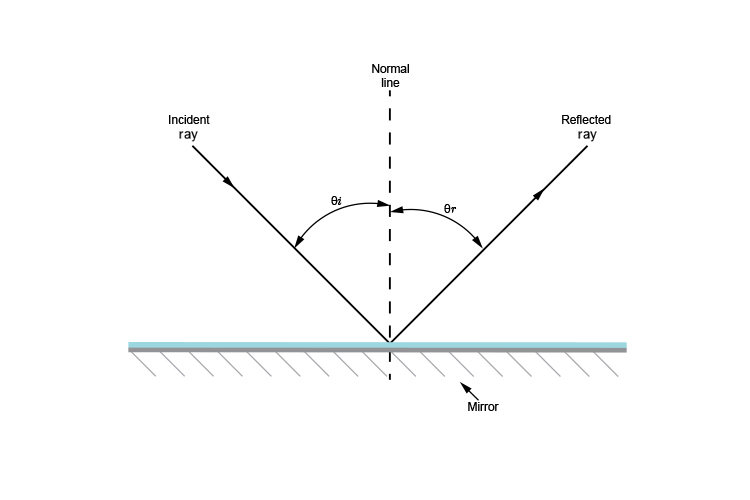So what happens when the mirror is tilted?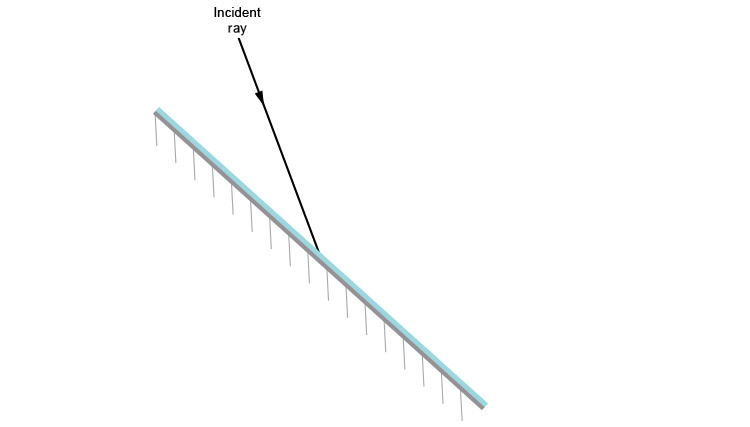In order to find the reflected ray you must first draw the normal line which is a line drawn perpendicular (at 90°) to the mirror at the point where the ray of light touches the mirror.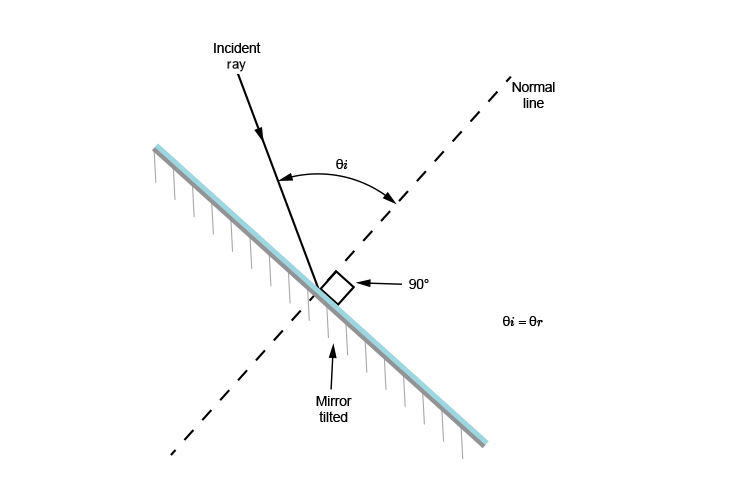We can now draw the reflected ray because we know the angle of incidence (thetai) is equal to the angle of refraction (thetar).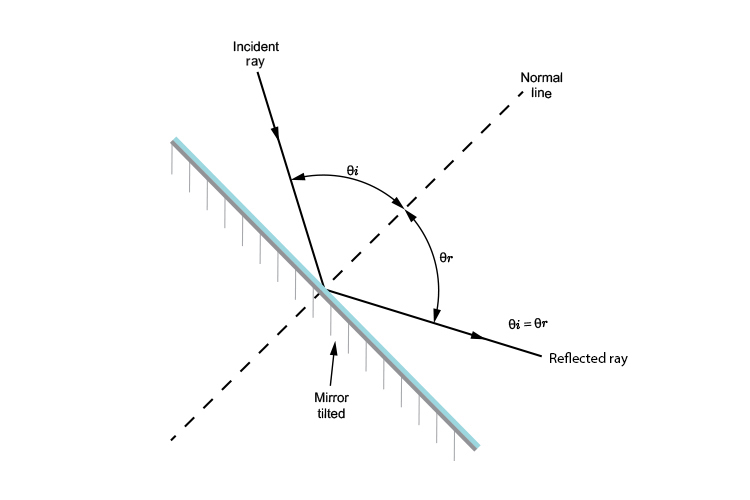We now have a completed ray diagram.

NOTE: There is an alternative way to find the reflected ray with flat mirrors. We can measure the angle between the incident ray and the flat mirror surface, and this will be the same on the other side.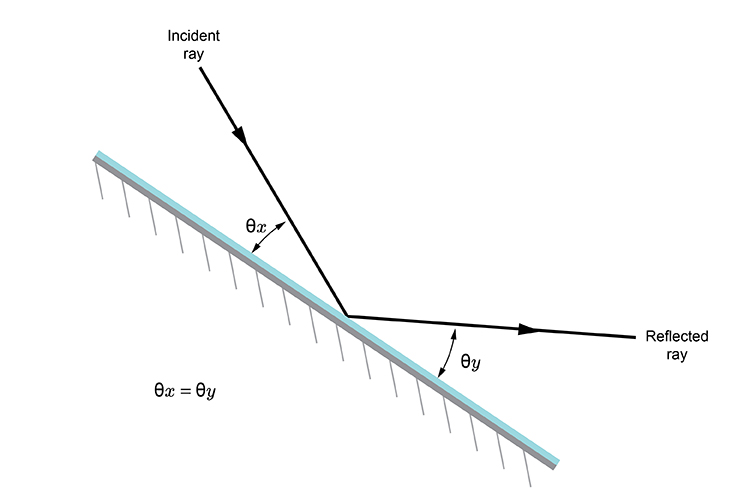However this technique can not be used with convex or concave mirrors so we advise using the normal line method for all mirrors. It's easier to remember one method.

## Conclusion

The normal line helps us locate the angle at which the angle of incidence equals the angle of reflection.